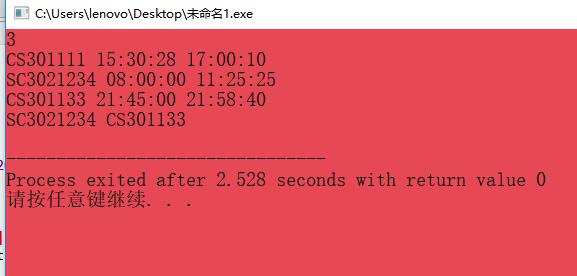2018-09-26 13:35

# 一道ACM题的题目部分测试不能通过

At the beginning of every day, the first person who signs in the computer room will unlock the door, and the last one who signs out will lock the door. Given the records of signing in's and out's, you are supposed to find the ones who have unlocked and locked the door on that day.

Input Specification:
Each input file contains one test case. Each case contains the records for one day. The case starts with a positive integer M, which is the total number of records, followed by M lines, each in the format:

ID_number Sign_in_time Sign_out_time
where times are given in the format HH:MM:SS, and ID_number is a string with no more than 15 characters.

Output Specification:
For each test case, output in one line the ID numbers of the persons who have unlocked and locked the door on that day. The two ID numbers must be separated by one space.

Note: It is guaranteed that the records are consistent. That is, the sign in time must be earlier than the sign out time for each person, and there are no two persons sign in or out at the same moment.

Sample Input:
3
CS301111 15:30:28 17:00:10
SC3021234 08:00:00 11:25:25
CS301133 21:45:00 21:58:40
Sample Output:
SC3021234 CS301133

``````
#include <iostream>
#include <string.h>
using namespace std;

struct stu
{
string num;     //学号
int c;          //c成绩
int m;          //m成绩
int e;          //e成绩
int a;          //平均成绩
int best;       //最好成绩
int bs;         //最好科目
int bR = 1;     //best rank
}list;

int main ()
{
int n, m;
cin >> n >> m;
for (int i = 0; i < n; i++)
{
int tmax = 0;   //暂存最大数
//int bs;
cin >> list[i].num;
cin >> list[i].c;
if (list[i].c > tmax)
{
tmax = list[i].c;
list[i].bs = 1;
}
cin >> list[i].m;
if (list[i].m > tmax)
{
tmax = list[i].m;
list[i].bs = 2;
}
cin >> list[i].e;
if (list[i].e > tmax)
{
tmax = list[i].e;
list[i].bs = 3;
}
list[i].a = int((list[i].c + list[i].m + list[i].e) / 3.0 + 0.5);
if (list[i].a >= tmax)
{
tmax = list[i].a;
list[i].bs = 4;
}
list[i].best = tmax;
}
for (int i = 0; i < m; i++)
{
int flag = 0;
string cn;    //需要检测的学号
cin >> cn;
for (int j = 0; j < n; j++)
{
if (cn == list[j].num)
{
int b = list[j].best;     //最好成绩
int s = list[j].bs;       //最好的科目
char c;
if (s == 1)
{
for (int k = 0; k < n; k++)
{
if (j != k && list[k].c > b)
list[j].bR += 1;
}
c = 'C';
}
if (s == 2)
{
for (int k = 0; k < n; k++)
{
if (j != k && list[k].m > b)
list[j].bR += 1;
}
c = 'M';
}
if (s == 3)
{
for (int k = 0; k < n; k++)
{
if (j != k && list[k].e > b)
list[j].bR += 1;
}
c = 'E';
}
if (s == 4)
{
for (int k = 0; k < n; k++)
{
if (j != k && list[k].a > b)
list[j].bR += 1;
}
c = 'A';
}
cout << list[j].bR << ' ' << c << endl;
flag = 1;
break;
}
}
if (!flag)
cout << "N/A" << endl;
}

}

``````
``````

``````
• 写回答
• 关注问题
• 收藏
• 邀请回答

#### 2条回答默认 最新

•扫眉 2018-09-26 13:46
已采纳这边注释一下

`````` #include <iostream>
#include <cstdio>
#include <cstring>

int main(void)
{
setvbuf(stdin, new char[1 << 20], _IOFBF, 1 << 20);
setvbuf(stdout, new char[1 << 20], _IOFBF, 1 << 20);
int m;
scanf("%d", &m);
char id_open, id_close, t_open[] = "23:59:59", t_close[] = "00:00:00";
char id, arrive, depart;
for (int i = 0; i < m; i++) {    //开始处理m组数据
scanf("%s %s %s", id, arrive, depart);
if (strcmp(t_open, arrive) > 0) {  //开始比较
strcpy(id_open, id);
strcpy(t_open, arrive);
}
if (strcmp(t_close, depart) < 0) {
strcpy(id_close, id);
strcpy(t_close, depart);
}
}
printf("%s %s\n", id_open, id_close);
return 0;
}
``````
已采纳该答案
打赏 评论
•扫眉 2018-09-26 13:38

我觉得这道题考察以时间为核心的事件模拟
时间复杂度方面时间复杂度O(n)，空间复杂度O(1）
我的思路如下：
1.读入M。
2.进行M次循环。设置开门时间char t_open[] = 235959，关门时间char t_close[] = 000000。开门人char id_open，关门人id_close。
1.读入身份号码，上班时间和下班时间。
2.使用strcmp将上班时间与t_open进行比较，如果上班时间小于t_open，那么strcpy(t_open,上班时间)。strcpy(id_open, id)。
3.使用strcmp将下班时间与t_close进行比较，如果下班时间大于t_close，那么strcpy(t_close, 下班时间)。strcpy(id_close, id)。
3.输出结果。
这是我的代码，可以参考一下

``````#include <iostream>
#include <cstdio>
#include <cstring>

int main(void)
{
setvbuf(stdin, new char[1 << 20], _IOFBF, 1 << 20);
setvbuf(stdout, new char[1 << 20], _IOFBF, 1 << 20);
int m;
scanf("%d", &m);
char id_open, id_close, t_open[] = "23:59:59", t_close[] = "00:00:00";
char id, arrive, depart;
for (int i = 0; i < m; i++) {
scanf("%s %s %s", id, arrive, depart);
if (strcmp(t_open, arrive) > 0) {
strcpy(id_open, id);
strcpy(t_open, arrive);
}
if (strcmp(t_close, depart) < 0) {
strcpy(id_close, id);
strcpy(t_close, depart);
}
}
printf("%s %s\n", id_open, id_close);
return 0;
}

``````
``````
``````

希望对你有帮助！

打赏 评论